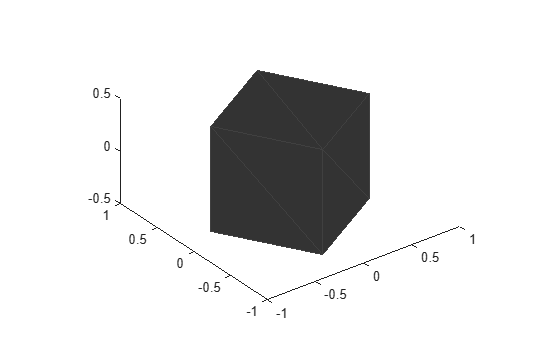# rotate

## Syntax

``rotatedMesh = rotate(mesh,orient)``

## Description

example

````rotatedMesh = rotate(mesh,orient)` rotate the `mesh` object by an orientation, `orient`. ```

## Examples

collapse all

Create an `extendedObjectMesh` object and rotate the object.

Construct a cuboid mesh.

`mesh = extendedObjectMesh('cuboid');`

Rotate the mesh by 30 degrees around the z axis.

`mesh = rotate(mesh,[30 0 0]);`

Visualize the mesh.

`ax = show(mesh);`## Input Arguments

collapse all

Extended object mesh, specified as an `extendedObjectMesh` object.

Description of rotation for an object mesh, specified as:

• 3-by-3 orthonormal rotation matrix

• `quaternion`

• 1-by-3 vector, where the elements are positive rotations in degrees about the z, y, and x axes, in that order.

## Output Arguments

collapse all

Rotated object mesh, returned as an `extendedObjectMesh` object.

## Version History

Introduced in R2022a